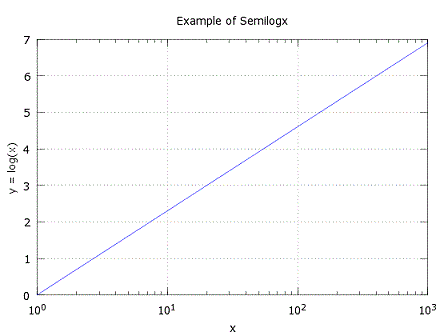# loglog - logarithmic plot

In this example we are going to demonstrate how to use the 'loglog' function included in Matlab to produce non-linear plots.

This term refers to the fact that the plot is logarithmically scaled in both axes. There are other functions such as 'semilogx' and 'semilogy' which have one axis in linear scale and the other axis in logarithmic scale.

### 1.- Create a plot using a logarithmic scale for both the x-axis and the y-axis (loglog):

clear; clc; close all

t = 0 : 2*pi/360 : 2*pi;

% Define values along your x-axis
x = exp(t);
% Define values along your y-axis
y = 50 + exp(3*t);

% Plot your function with a wider line and grid the figure
loglog(x, y, 'LineWidth', 2)
grid

% Use a title for the figure
title('Demonstration of logarithmic plots')

% Label your x-axis with a double line.
% Note the special characters

xlabel([{'e^{t}'}; {'0 \leq t \leq 2\pi'}])

ylabel('50 + e^{3t}')

The produced figure is:### 2.- Create a plot with a logarithmic scale for the x-axis and a linear scale for the y-axis (semilogx):

% clear memory data and command window
clear; clc; close all

x = 0 : 1000;
y = log(x);

% plot your log function on linear data
semilogx(x, y)

% define values of axes and grid
axis([1 1000 0 7])
grid

title('Example of Semilogx')
xlabel('x')
ylabel('y = log(x)')

The resulting figure is### 3.- Create a plot with a logarithmic scale for the y-axis and a linear scale for the x-axis (semilogy):

clear; clc; close all

% now your data along the x-axis is linear
x = 0 : 0.5 : 10;

% your function is now exponential
y = exp(x);

% see the plot
semilogy(x, y)
plot

title('Example of Semilogy')
xlabel('x')
legend('y = e^x')

The plot isFrom 'loglog' to home

From 'loglog' to '2D Plot Menu'